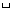Next: B.3 ``Substring Array'' Convention Up: B Proposed Binary Table Previous: B.1 ``Variable Length Array''

# B.2 ``Multidimensional Array'' Convention

It is anticipated that binary tables will need to contain data structures more complex that those describable by the basic notation. Examples of these are  multidimensional arrays and nonrectangular data structures. Suitable conventions may be defined to pass these structures using some combination of keyword/value pairs and table entries to pass the parameters of these structures.

One case, multidimensional arrays, is so common that it is prudent to describe a simple convention. The ``Multidimensional array'' convention consists of the following: any column with a dimensionality of 2 or larger will have an associated character  keyword TDIMn ='(l,m,n...)' where l, m, n,...are the dimensions of the array. The data is ordered such that the array index of the first dimension given (l) is the most rapidly varying and that of the last dimension given is the least rapidly varying. The size implied by the TDIMn keyword will equal the element count specified in the TFORMn keyword. The adherence to this convention will be indicated by the presence of a TDIMn keyword in the form described above.

A character string  is represented in a binary table by a one-dimensional character array, as described under ``Character'' in the list of datatypes in §8.3.3.1 (``Main Data Table ''). For example, a Fortran 77 CHARACTER*20 variable could be represented in a binary table as a character array declared as TFORMn = `'20A'`. Arrays of character strings, i.e., multidimensional character arrays, may be represented using the TDIMn notation. For example, if TFORMn = `'60A'` and `TDIMn = '(5,4,3)'`, then the entry consists of a 4 × 3 array of strings of 5 characters each. (Variable length character strings are allowed by the convention described in Appendix B.3. One dimensional arrays of strings should use the convention in Appendix B.3 rather than the ``Multidimensional Array'' convention.)

This convention is optional and will not preclude other conventions. This convention is not part of the binary table definition.Next: B.3 ``Substring Array'' Convention Up: B Proposed Binary Table Previous: B.1 ``Variable Length Array''

5/13/1999